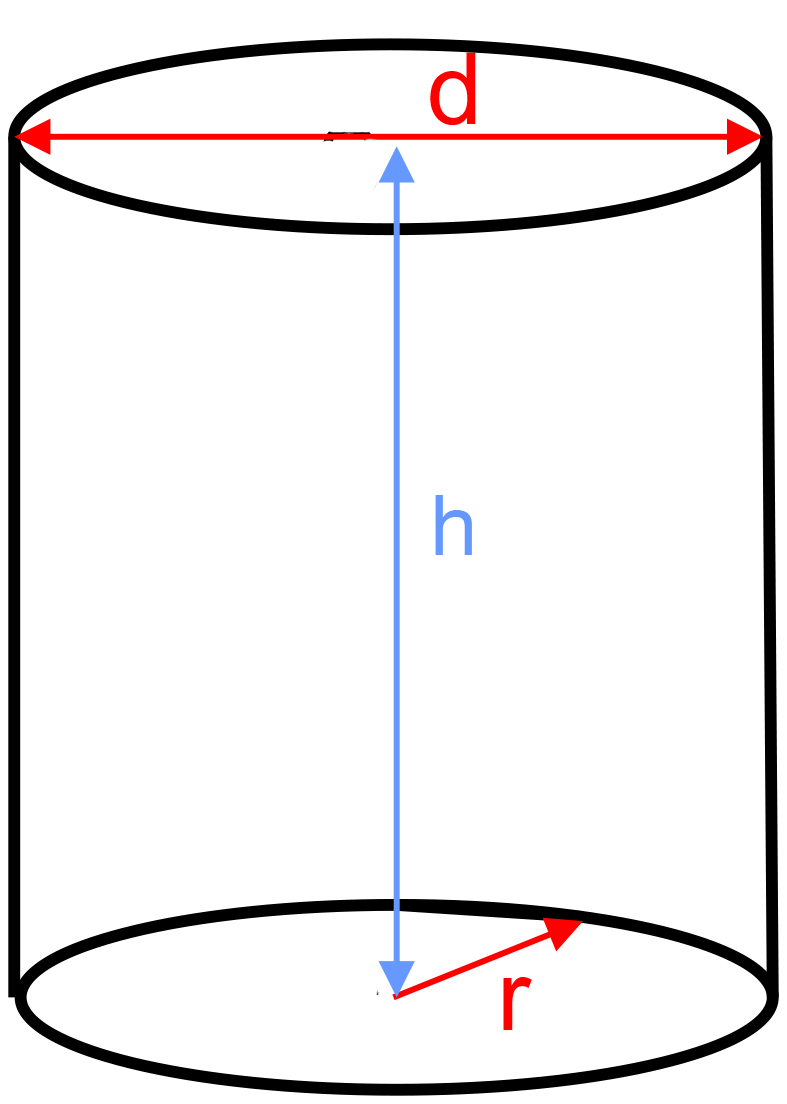﻿ Cylinder - circular flat: - online výpočet - FORMIAX

# Cylinder (circular flat) - surface and volumeS = 2πrv + 2πr2

V = πr2v

S = surface area

V = volume

r = radius = 1/2 d

d = diameter

h = height

WHAT IS IT ?
Cylinder is solid object with two identical flat ends that are circular or elliptical and one curved side. It has the same cross-section from one end to the other.

CALCULATION:

Enter unit e.g.: inch

Enter perimeter

Enter height

Round to number of decimals places

YOU MIGHT BE INTERESTED: